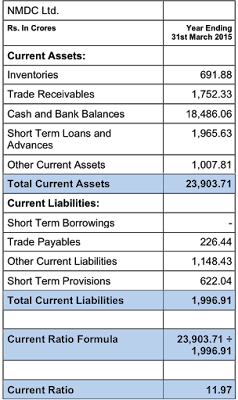## Friday, September 23, 2016

### Current Ratio - Formula

#### Current Ratio Formula:Formula for Calculation of Current Ratio

#### Meaning:

Current Ratio is the ratio or proportion between current assets and current liabilities. Current assets mean assets that can be expected to get converted into cash within a year and current liabilities are those that mature within 12 months. It is a measure of the business’s liquidity or the ability to meet short-term obligations.

#### Desirable Number:

Current ratio of two is minimum stipulated. Any number above means additional safety. A number below 2 indicates weakness.

Example:
Here we present the example of calculating the current ratio of NMDC Ltd., as per the balance sheet on 31st March 2015.Example Showing Current Ratio Calculation of NMDC Ltd.